Physics Department                [Return to Examples]

# Thermo Example 4© 2000 D.P. Maloney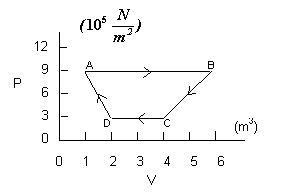The cycle in the P-V diagram at the right

is for an ideal gas.  Find the work done,

on or by, the gas during each of the four

stages of the process, and the net work

done in the cycle.

Given: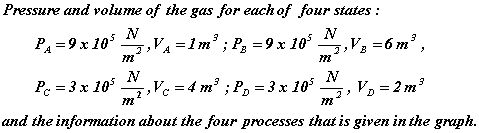Unknown: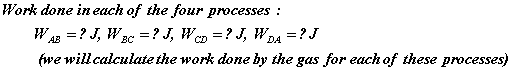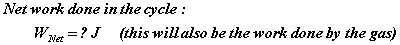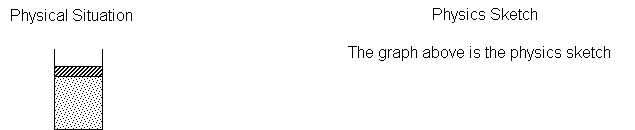Physical Principles and/or Ideas:    Relations for work done on or by an ideal gas in an isobaric process and for other processes diagrammed on a P-V graph.

Solution:         The work done by, or on, an ideal gas is computed by taking the product of the pressure and the change in volume.  That is the general prescription for determining the work, but this prescription can only be applied directly to isobaric processes.  For other types of processes, the calculation is more involved.

The first process in this problem is an isobaric one so the process of computing the work done is straightforward.  As we see in the graph, the pressure is constant (that is what isobaric means) for process AB.  The volume changes from 1 m3 to 6 m3 so the work done by the gas is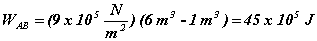Notice that this is positive, meaning that this work was done by the gas, not on the gas.  This just reflects the fact that a gas can expand if the situation is appropriate, but something must act on a gas to make it contract.

Process BC is not an isobaric process since the pressure changes.  For BC, the volume also changes.  This time we cannot simply multiply the change in volume by one pressure since the change did not occur at one pressure.  But notice that calculating the “area” on the graph under the line involves a computation where pressure and volume are going to be multiplied, consequently, that will give us work.  But notice also that we are not calculating an area in the sense of length times width, but rather an “area” in the sense of the amount of surface in a particular region of the graph.  For process BC, the region we want has two parts:  a rectangle and a triangle (see the graph below).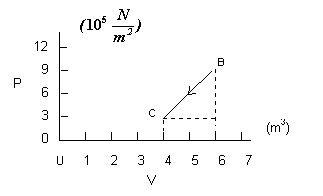The “area” of the rectangular region is: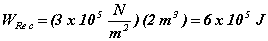The “area” of the triangular region is: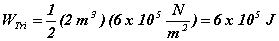So the work done by the gas in process BC is: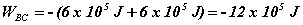It is negative because work must be done on a gas to compress it.

Process CD is an isobaric process, so we can calculate the work from: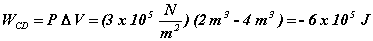Finally, the work done in process DA is determined by finding the “area” under the line DA in the graph.  Again, the relevant region is composed of a rectangular region and a triangular region.  The “area” of the rectangular region is: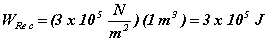The “area” of the triangular region is: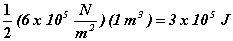So the work done by the gas in process DA is: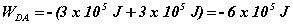The net work done in the whole cycle is: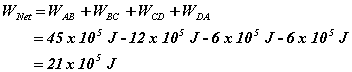This is positive, so that means the work is done by the gas.

Suppose there are 3 moles of gas in this situation.  How would you determine the initial temperature of the gas?

Other Thermo Examples:    1     2     3     4     5
[Examples Homepage]      [Physics Homepage]      [IPFW Homepage]